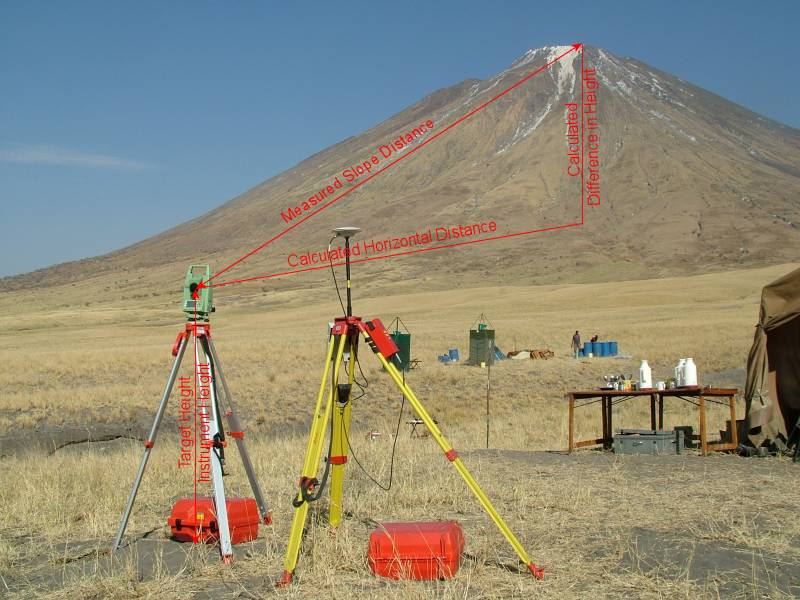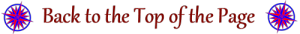# Measuring the Height of Ol Doinyo Lengai

Introduction

The measurement of position for the Gravity Meter survey using DGPS by both the Survey and Geosciences Fires meant that accurate height information was available at the Lengai base camp.  The opportunity was taken to determine the height of Ol Doinyo Lengai by transferring this height information using the high accuracy Leica TPS1200 Total Station that was being used to produce a map of the crater of Ol Doinyo Lengai using its reflectorless measurement capabilities.

## Method

The location and height of the ground point below the yellow tripod on the right in Figure 1 was determined by DGPS from the ISG Earth Station at Malindi and DGPS was used to transfer the height to the ground point below the aluminium tripod on the left.  From the observations taken to determine the locations and heights for the Gravity Meter readings the precision of this height on the earth’s surface is known to 0.1 metres.

Vertical Angles and the Slope Distance were taken between this point and the TPS1205 located at the top of Ol Doinyo Lengai.  The values for the Difference in Height between the two instruments was calculated using the right angled triangle with the Measured Slope Distance as the hypotenuse.  The “line of sight” is not a straight line as it refracted by the atmosphere that it passes through and influenced by the curvature of the earth.  These were taken into account and “corrected” for to determine the actual Difference in Height.  This value is the difference to the units on the tripods so the Target and Instrument Heights were applied to determine the actual difference in height between the ground points.Figure 1 Equipment setup and method of determining the height of Ol Doinyo Lengai. See text for discussion.

## Results

The height of Ol Doinyo Lengai determined by these techniques is:

Ellipsoidal (or GPS) Height 2962.2 metres.

Orthometric Height (height above mean sea level) 2951.6 metres.

Hugh Anderson  MRICS MInstCES BA(Open), Survey Fire Leader